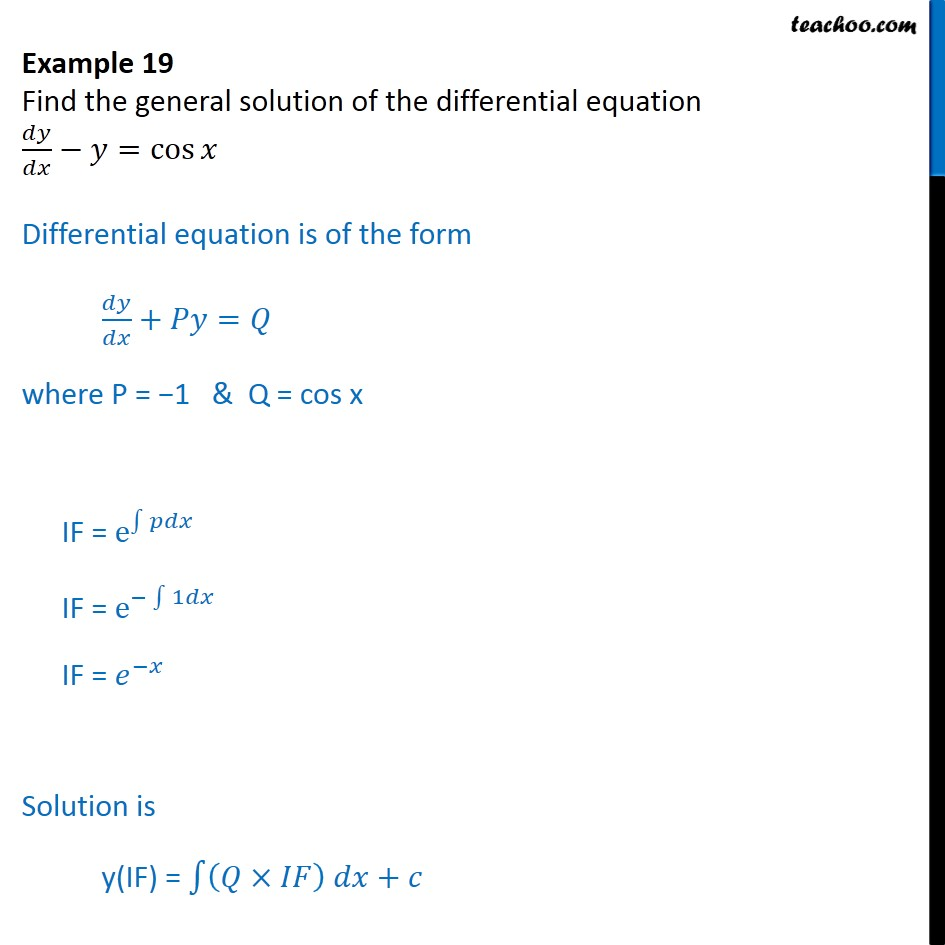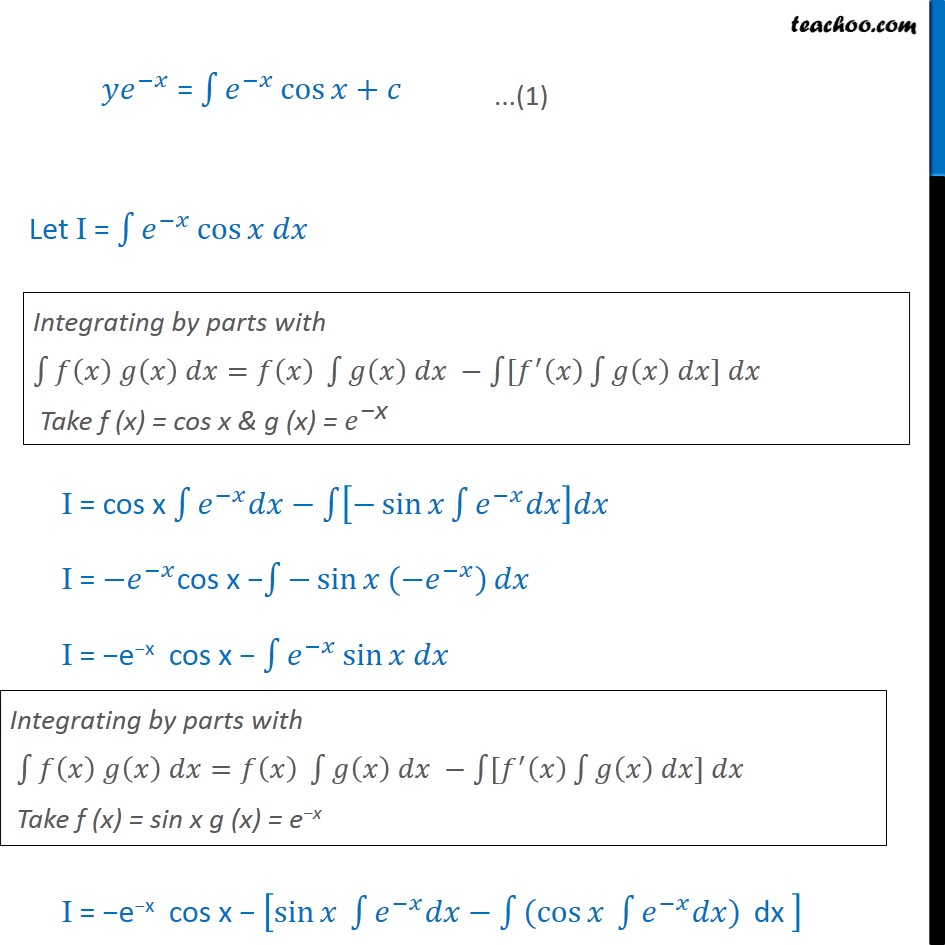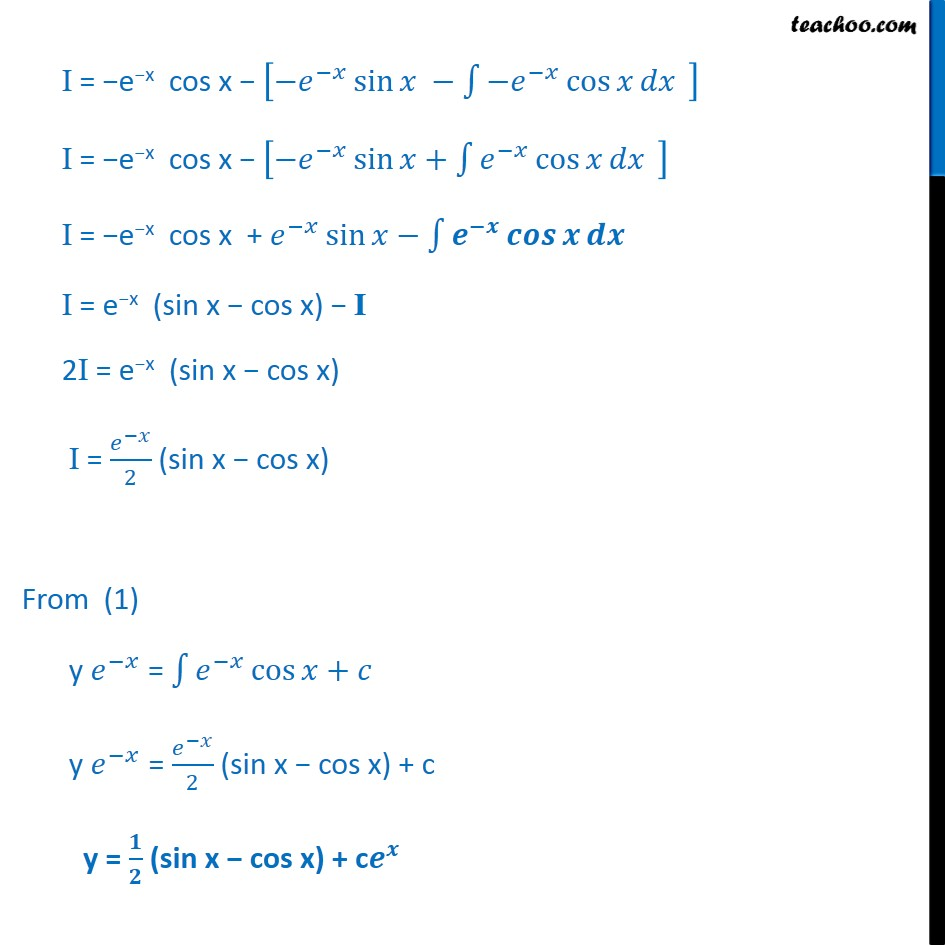Examples

Chapter 9 Class 12 Differential Equations
Serial order wiseLearn in your speed, with individual attention - Teachoo Maths 1-on-1 Class

### Transcript

Example 14 Find the general solution of the differential equation 𝑑𝑦/𝑑𝑥−𝑦=cos⁡𝑥 Differential equation is of the form 𝑑𝑦/𝑑𝑥+𝑃𝑦=𝑄 where P = −1 & Q = cos x IF = e^∫1▒𝑝𝑑𝑥 IF = e^(−∫1▒1𝑑𝑥) IF = 𝑒^(−𝑥) Solution is y(IF) = ∫1▒〖(𝑄×𝐼𝐹) 𝑑𝑥+𝑐〗 𝑦𝑒^(−𝑥) = ∫1▒𝑒^(−𝑥) cos⁡〖𝑥+𝑐〗 Let I = ∫1▒𝑒^(−𝑥) cos⁡〖𝑥 𝑑𝑥〗 Integrating by parts with ∫1▒〖𝑓(𝑥) 𝑔(𝑥) 𝑑𝑥=𝑓(𝑥) ∫1▒〖𝑔(𝑥) 𝑑𝑥 −∫1▒〖[𝑓^′ (𝑥) ∫1▒〖𝑔(𝑥) 𝑑𝑥] 𝑑𝑥〗〗〗〗 Take f (x) = cos x & g (x) = 𝑒^"−x" I = cos x ∫1▒〖𝑒^(−𝑥) 𝑑𝑥〗− ∫1▒[−sin⁡〖𝑥∫1▒〖𝑒^(−𝑥) 𝑑𝑥〗〗 ]𝑑𝑥 I = 〖−𝑒〗^(−𝑥)cos x −∫1▒〖−sin⁡〖𝑥 (−𝑒^(−𝑥) 〗)〗 𝑑𝑥 I = −e−x cos x − ∫1▒〖𝑒^(−𝑥) sin⁡〖𝑥 𝑑𝑥〗 〗. Integrating by parts with ∫1▒〖𝑓(𝑥) 𝑔(𝑥) 𝑑𝑥=𝑓(𝑥) ∫1▒〖𝑔(𝑥) 𝑑𝑥 −∫1▒〖[𝑓^′ (𝑥) ∫1▒〖𝑔(𝑥) 𝑑𝑥] 𝑑𝑥〗〗〗〗 Take f (x) = sin x g (x) = e−x I = −e−x cos x − [sin⁡〖𝑥 ∫1▒〖𝑒^(−𝑥) 𝑑𝑥〗−∫1▒〖(cos〗⁡〖𝑥 ∫1▒〖𝑒^(−𝑥) 𝑑𝑥〗)〗 "dx " 〗 ] I = −e−x cos x − [−𝑒^(−𝑥) sin⁡〖𝑥 −∫1▒〖−𝑒^(−𝑥) cos⁡𝑥 𝑑𝑥〗 " " 〗 ] I = −e−x cos x − [−𝑒^(−𝑥) sin⁡〖𝑥+∫1▒〖𝑒^(−𝑥) cos⁡𝑥 𝑑𝑥〗 " " 〗 ] I = −e−x cos x + 𝑒^(−𝑥) sin⁡〖𝑥−∫1▒〖𝒆^(−𝒙) 𝒄𝒐𝒔⁡𝒙 𝒅𝒙〗 " " 〗 I = e−x (sin x − cos x) − I 2I = e−x (sin x − cos x) I = 𝑒^(−𝑥)/2 (sin x − cos x) From (1) y 𝑒^(−𝑥) = ∫1▒〖𝑒^(−𝑥) cos⁡〖𝑥+𝑐〗 〗 y 𝑒^(−𝑥) = 𝑒^(−𝑥)/2 (sin x − cos x) + c y = 𝟏/𝟐 (sin x − cos x) + c𝒆^𝒙